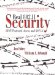# Nonce Selection

 The idea of the nonce values is that they are used only once with a given key. The important thing is that this should hold true even when the mobile device or access point is restarted or even if a Wi-Fi LAN adapter card is moved from one laptop to another. The combination of key and MAC address should never use the same nonce value twice. If all equipment had a calendar/clock (and could guarantee it was correct), this problem can be easily solved. For example, the nonce value could be initialized after startup, to the value of Network Time (number of seconds since midnight, Dec 31st, 1899). Providing, on average, you don't need a nonce more than once per second, you will always get a value that has not been used before (unless time starts to run backwards, which seems unlikely). However, not all systems have a calendar clock and the accuracy can't be guaranteed anyway. Therefore, the nonce is created from a large counter that is initialized to a random value at start up. The idea is that if the counter is large enough, the probability that you will ever reuse the same range of values is so small as to be unimportant. This counter is 256 bits long. Suppose that an access point starts up and sets its 256-bit nonce counter to a perfectly random value. Then suppose that the access point generates ten thousand nonces before the next restart (incrementing the counter each time). New nonces are needed only when the group keys are refreshed and when a mobile device joins the network. Given the size of the nonce counter, the probability that the access point will reinitialize the counter to one of the 10,000 values it has just used is an embarrassingly low 1 in 10-70 probably about the same probability that time will start to run backwards. There is a problem, however, because this analysis relies on generation of a perfectly random number, which can also be difficult to do. Because of the difficulty of generating perfect random numbers, RSN and WPA specify a way to generate the starting value for the nonce counter by using a pseudorandom number generator. The formula is written using the function PRF-256. This is the random number generator function that is also used for key derivation. Operation of this function is described in the following section on key computation. Here is the formula for computing the start value of the nonce counter: Starting nonce = PRF-256(Random Number, "Init Counter", MAC || Time) where: Random Number is the best random number the equipment can make."Init Counter" is a literal string.MAC || Time is the MAC address of the device concatenated with the best guess at Network Time (if known). If an attacker is going to base an attack on finding duplicate nonce values, he is going to have to wait a long time!Real 802.11 Security: Wi-Fi Protected Access and 802.11i
ISBN: 0321136209
EAN: 2147483647
Year: 2005
Pages: 151

Similar book on Amazon